# 1-生成模型

## 1-1 生成模型与判别模型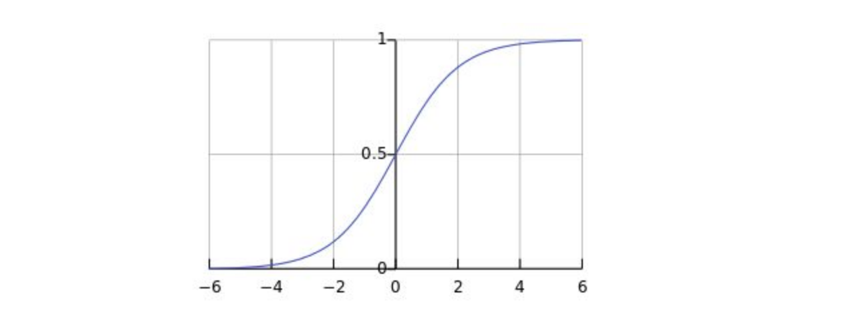P(Y|X)=P(X,Y)P(X)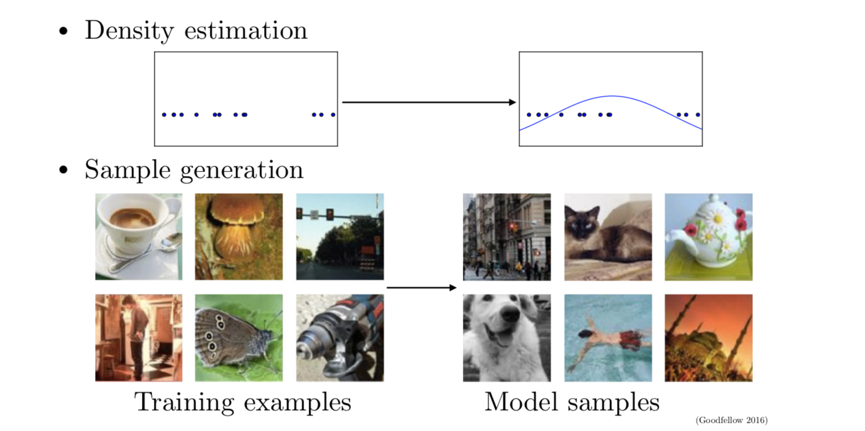## 1-2 为什么学习生成模型？

1. 能生成高维的数据或者复杂的概率分布，且高维数据分布在数学和工业界都扮演着重要的作用
2. 还可以为强化学习做一定准备
3. 对于缺失数据较多的场景，可以用来生成更多的样例数据，是当前用来解决信息缺失的最好方式。

Next Video Frame Prediction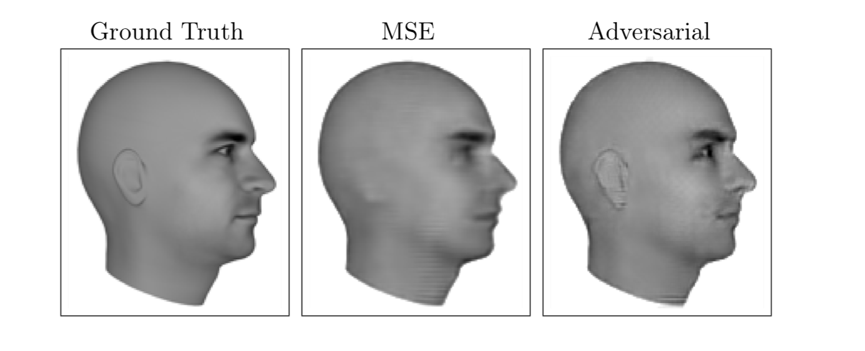Single Image Super-Resolution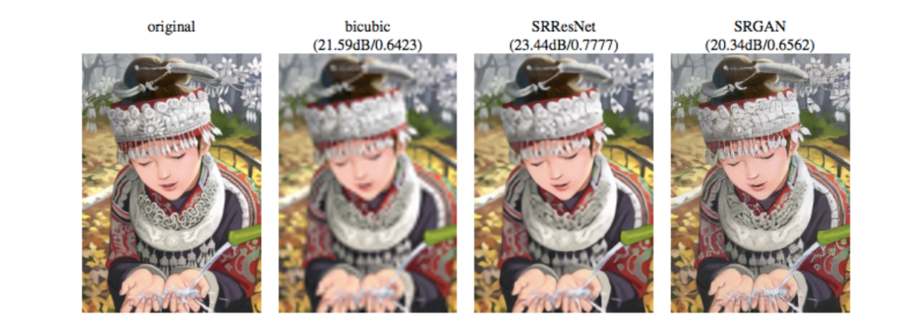Image to Image Translation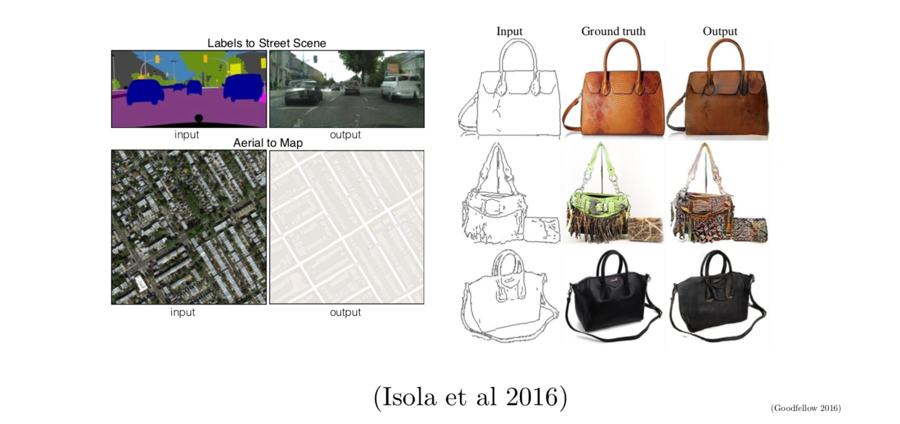## 1-3 生成模型原理—似然原理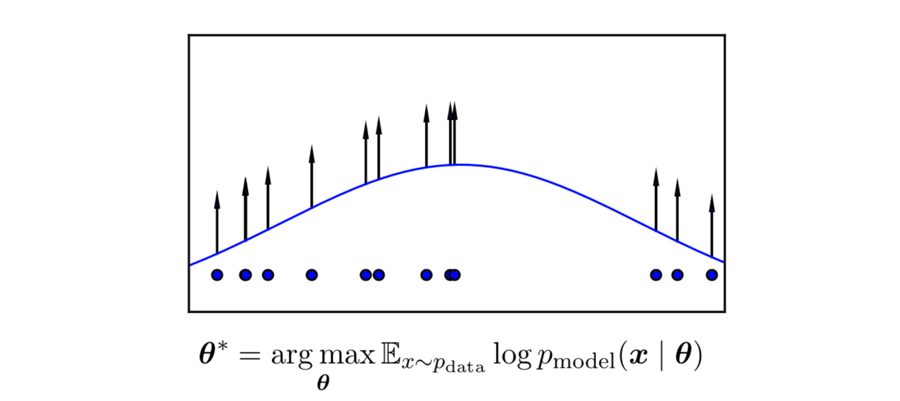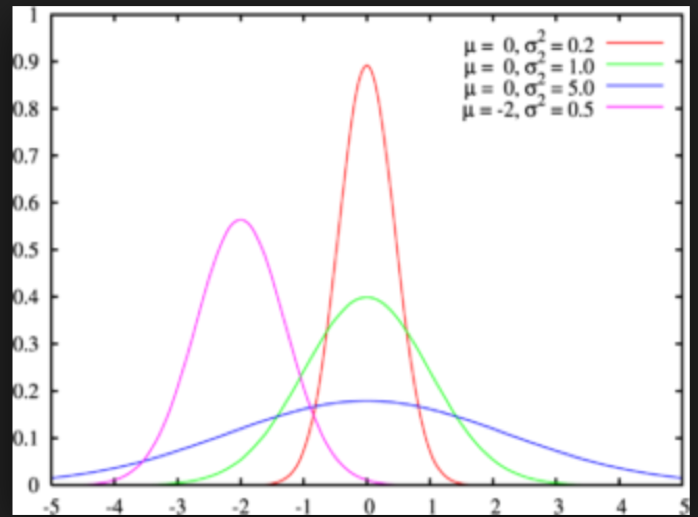# 2-生成式对抗网络

## 2-1 生成式对抗网络工作原理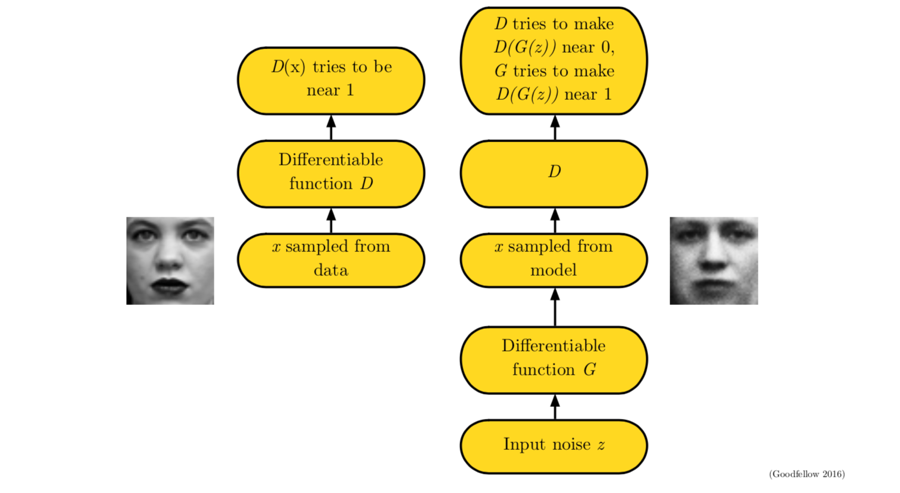GAN是一种structured probabilistic model，具体介绍在deep learning这本书的第16章有。GAN是一个有向图模型，它的每一个隐变量都在影响观测变量。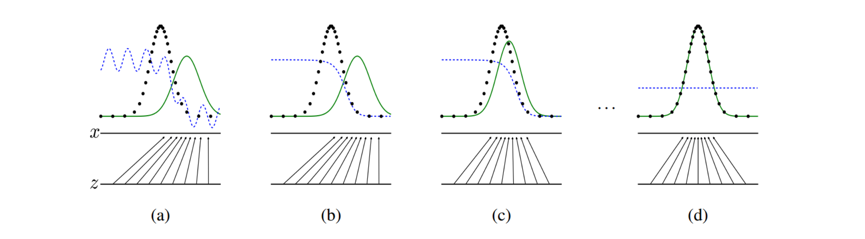• 判别器D(x)$D(x)$独自训练自己，希望能分辨出真实的数据分布和生成器给的数据分布
• 生成器G(z)$G(z)$也训练自己，希望以假乱真，让判别器判别不出到底哪个是真，哪个是假

G(z)$G(z)$D(x)$D(x)$它们的区别主要在于输入的数据和参数：θ(G),θ(D)$\theta^{(G)},\theta^{(D)}$.

• 举个例子，这个过程就像是一个画画的老师在教，或者说监督学生画画一样，老师就是对抗的部分，学生就是生成部分。老师手上有一副真的蒙娜丽莎，学生手上有一副蒙娜丽莎的赝品，学生在模仿赝品画蒙娜丽莎，然后给老师看，老实说你这里画得不像，重来，然后不断改进，直到学生画得蒙娜丽莎老师分辨不出来到底是真是假是，训练停止。

x=G(z;θ(G))

GAN的训练过程也是使用SGD，或者其他的优化算法来做优化，一般使用minibatch，值得注意的是，我们可以让其中一个先跑一下，一般让生成部分先跑， 使得生成的效率更高一点。

## 2-2 判别器的损失函数

ExpdatalogD(x)

D(x)=1   when  xpdata(x)

Ezpmodellog(1D(G(z)))
D(x)=0   when xpmodel(G(z))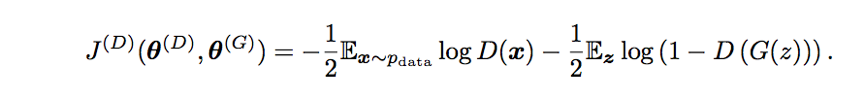## 2-3 生成器损失定义1（minimax）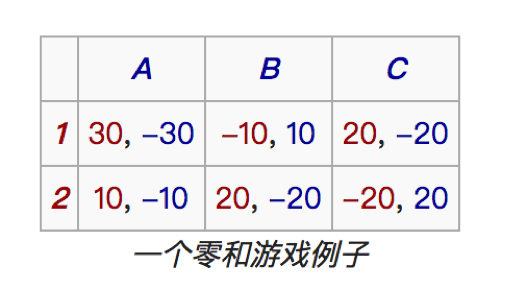J(G)=J(D)

## 2-4 生成器损失定义2：Non-Saturating Game

minimax的缺点就是生成器和判别器之间的损失函数直接关联，因此我们可以把他们的联系弄断，就变成了如下的样子：## 2-5 第三种策略：极大似然估计

### 2-5-1 KL 散度的定义E表示期望，因为离散型和连续型的随机变量的期望计算方法不一样，对于离散型随机变量的KL散度计算公式如下：

DKL(P||Q)=ip(i) logp(i)q(i)

DKL(P||Q)=+p(x) logp(x)q(x)

### 2-5-2 KL散度的推导

KL散度可以从极大似然估计中推导出来，而极大似然估计又是基于极大似然原理的，极大似然原理可以表示为：选择导致某“结果“发生可能性最大的原因，作为似然“原因“。说白了就是选择合适的参数使得某一事件发生的可能性最大。

L(θ)=f(y1|θ)f(y2|θ)f(yN|θ)

InL(θ)=In f(y1|θ)+In f(y2|θ)++In f(yN|θ)=iNIn f(yi|θ)
N$N \rightarrow \infty$时，几乎处处有：
1NiNIn f(yi|θ)=EIn f(Y|θ)

EIn f(Y|θ)=g(y) Inf(y|θ)dy

EIn g(y)E In f(y|θ)=g(y)In g(y)dy g(y) In f(y|θ)dy=KL(g|f)=g(y) Ing(y)f(y|θ)dy

### 2-5-3 利用KL散度度量生成分布与真实分布的相似性

GAN的生成器本质上是想使生成的函数尽可能和真实分布相似，以使得判别器没办法判断某一张图片是生成器生成的还是真实分布中的，因为为生成器找到一个度量生成分布中的图片与真实分布中的图片的差异性，是一个非常关键的问题，而KL散度刚好可以度量两个分布的相似性，因此，可以利用极大似然估计建立生成器对应的似然函数为：

L(θ)=PG(xi,θ)

arg minθKL(Pdata|PG)=Pdata(x)InPdata(x)PG(x|θ)

# 3-GAN实现

reference